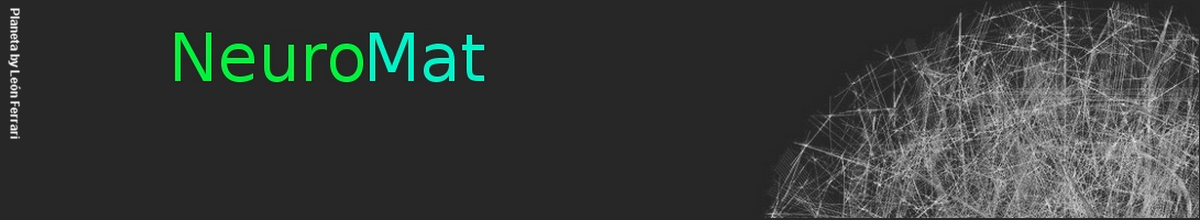### Stochastic chains with unbounded memory applied in neuroscience

Ricardo Felipe Ferreira is a mathematician who, during his doctoral research, was guided by the RIDC NeuroMat associated investigator Alexsandro Giacomo Grimbert Gallo (UFSCar). During the first semester of 2019, Ferreira defended his doctoral thesis, in which NeuroMat research is a highlight.

### Variance-Based Extragradient Methods with Line Search for Stochastic Variational Inequalities

Alfredo N. Iusem, Alejandro Jofré, Roberto I. Oliveira, and Philip Thompson

In this paper, we propose dynamic sampled stochastic approximated (DS-SA) extragradient methods for stochastic variational inequalities (SVIs) that are robust with respect to an unknown Lipschitz constant $L$. We propose, to the best of our knowledge, the first provably convergent robust SA method with variance reduction, either for SVIs or stochastic optimization, assuming just an unbiased stochastic oracle within a large sample regime. This widens the applicability and improves, up to constants, the desired efficient acceleration of previous variance reduction methods, all of which still assume knowledge of $L$ (and, hence, are not robust against its estimate). Precisely, compared to the iteration and oracle complexities of $\mathcal{O}(\epsilon^{-2})$ of previous robust methods with a small stepsize policy, our robust method uses a DS-SA line search scheme obtaining the faster iteration complexity of $\mathcal{O}(\epsilon^{-1})$ with oracle complexity of $(\ln L)\mathcal{O}(d\epsilon^{-2})$ (up to log factors on $\epsilon^{-1}$) for a $d$-dimensional space. This matches, up to constants, the sample complexity of the sample average approximation estimator which does not assume additional problem information (such as $L$). Differently from previous robust methods for ill-conditioned problems, we allow an unbounded feasible set and an oracle with multiplicative noise (MN) whose variance is not necessarily uniformly bounded. These properties are appreciated in our complexity estimates which depend only on $L$ and local variances or fourth moments at solutions. The robustness and variance reduction properties of our DS-SA line search scheme come at the expense of nonmartingale-like dependencies (NMDs) due to the needed inner statistical estimation of a lower bound for $L$. In order to handle an NMD and an MN, our proofs rely on a novel iterative localization argument based on empirical process theory.

The whole paper is available here.

### Modeling neuronal avalanches and long-range temporal correlations at the emergence of collective oscillations: Continuously varying exponents mimic M/EEG results

Leonardo Dalla Porta and Mauro Copelli

We revisit the CROS (“CRitical OScillations”) model which was recently proposed as an attempt to reproduce both scale-invariant neuronal avalanches and long-range temporal correlations. With excitatory and inhibitory stochastic neurons locally connected in a two-dimensional disordered network, the model exhibits a transition where alpha-band oscillations emerge. Precisely at the transition, the fluctuations of the network activity have nontrivial detrended fluctuation analysis (DFA) exponents, and avalanches (defined as supra-threshold activity) have power law distributions of size and duration. We show that, differently from previous results, the exponents governing the distributions of avalanche size and duration are not necessarily those of the mean-field directed percolation universality class (3/2 and 2, respectively). Instead, in a narrow region of parameter space, avalanche exponents obtained via a maximum-likelihood estimator vary continuously and follow a linear relation, in good agreement with results obtained from M/EEG data. In that region, moreover, the values of avalanche and DFA exponents display a spread with positive correlations, reproducing human MEG results.

### Packing arborescences in random digraphs

Carlos Hoppen, Roberto F. Parente and Cristiane M. Sato

We study the problem of packing arborescences in the random digraph D(n, p), where each possible arc is included uniformly at random with probability p = p(n). Let λ(D(n, p)) denote the largest integer λ ≥ 0 such that, for all 0 ≤ ≤ λ, we have −1 i=0 ( − i)|{v : din(v) = i}| ≤ . We show that the maximum number of arc-disjoint arborescences in D(n, p) is λ(D(n, p)) a.a.s. We also give tight estimates for λ(D(n, p)) depending on the range of p. Keywords: random graph, random digraph, edge-disjoint spanning tree, spanning tree, packing arborescence.

### Stochastic oscillations and dragon king avalanches in self-organized quasi-critical systems

Osame Kinouchi, Ludmila Brochini, Ariadne A. Costa, João Guilherme Ferreira Campos and Mauro Copelli

In the last decade, several models with network adaptive mechanisms (link deletion-creation, dynamic synapses, dynamic gains) have been proposed as examples of self-organized criticality (SOC) to explain neuronal avalanches. However, all these systems present stochastic oscillations hovering around the critical region that are incompatible with standard SOC. Here we make a linear stability analysis of the mean field fixed points of two self-organized quasi-critical systems: a fully connected network of discrete time stochastic spiking neurons with firing rate adaptation produced by dynamic neuronal gains and an excitable cellular automata with depressing synapses. We find that the fixed point corresponds to a stable focus that loses stability at criticality. We argue that when this focus is close to become indifferent, demographic noise can elicit stochastic oscillations that frequently fall into the absorbing state. This mechanism interrupts the oscillations, producing both power law avalanches and dragon king events, which appear as bands of synchronized firings in raster plots. Our approach differs from standard SOC models in that it predicts the coexistence of these different types of neuronal activity.

NeuroCineMat
Featuring this week: